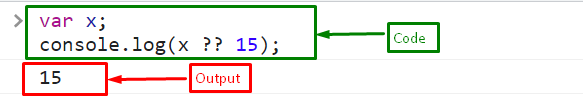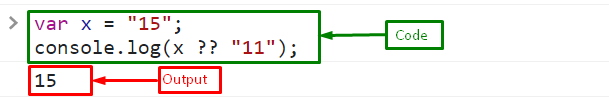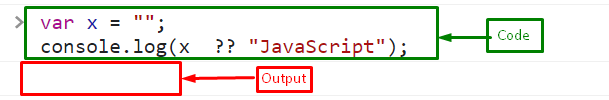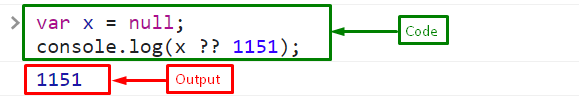# Double Question Mark in JavaScript

In JavaScript, there are multiple operators that are used to efficiently handle different scenarios. One such operator is the “double question mark” (??) or the “Null Coalescing Operator” introduced in ECMAScript 2020. This operator addresses setting the default values for “null” or “undefined” variables. JavaScript developers can use the double question mark operator to simplify and shorten their code.

This tutorial will demonstrate the double question mark “??” in JavaScript.

## What is a Double Question Mark “??” in JavaScript?

The “??” is a logical operator utilized for checking the “undefined” or “null” values. In “double question mark” (??), the first statement is evaluated and the right-hand side expression or “statement2” will be assigned if “statement1” or left-hand side expression is null or undefined.

Syntax

The given syntax is utilized for using a double question mark in JavaScript:

`expression1 ?? expression2`

### Example 1: Check the Value of a Declared Variable

Declare a variable “x” and use the “Null Coalescing Operator” to check the value of x. If it is null or undefined, then return a right-hand side or default value:

```var x;
console.log(x ?? 15);```

The output displays the default value or the right-hand side value because the variable “x” is undefined:### Example 2: Check the Value of an Initialized Variable

In this example, we will assign a value “15” to the variable “x” and then check the variable’s value using the “??” operator:

```var x = "15";
console.log(x ?? "11");```

As you can see that the output prints the value of “x” because the x is not null or undefined so the operator returns the left-hand side expression:### Example 3: Check Empty Variable

Here, variable “x” stores an empty string and we will check the value of “x” using a double question mark. It will print the empty string because x is not null or undefined so the null coalescing operator gives the left-hand side expression:

```var x = "";
console.log(x ?? "JavaScript");```

Output### Example 4: Check Variable Having “null” Value

If the variable stores “null”, then the null coalescing or “??” outputs the right-hand side expression:

```var x = null;
console.log(x ?? 1151);```

OutputThat was all about the “??” and its usage in JavaScript.

## Conclusion

The “double question mark” (??) is a logical operator similar to the OR operator. But the difference is that the logical OR operator evaluates falsy values while the double question mark operator only checks for null or undefined values. It helps to avoid lengthy conditional statements and simplify the code. This tutorial described the usage of double question mark “??” in JavaScript.